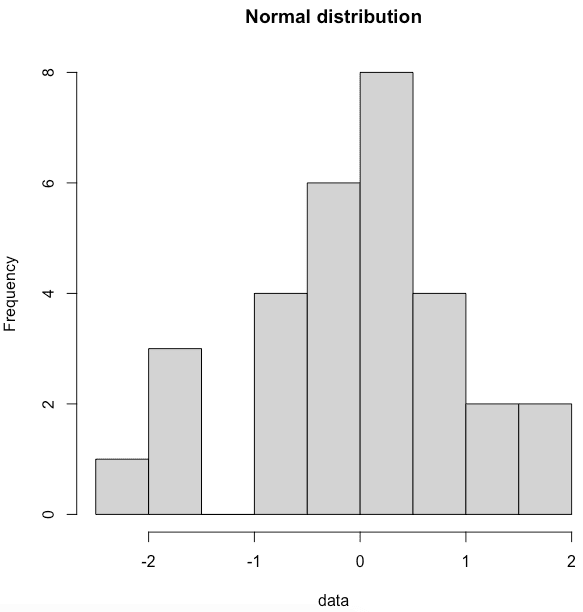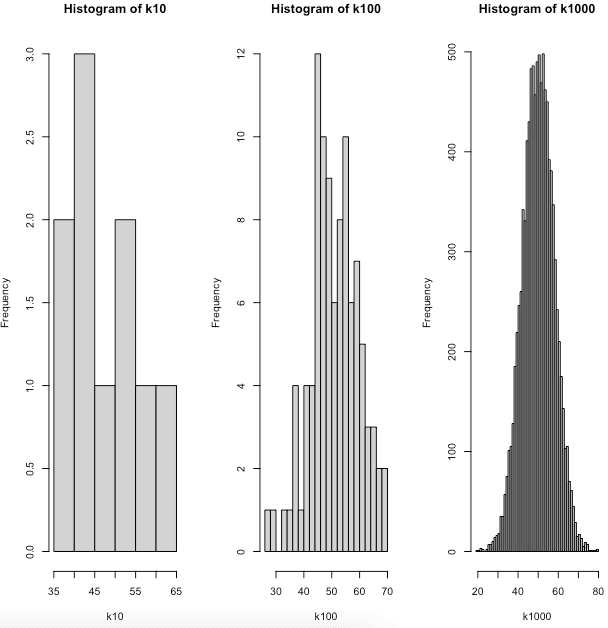# Random Normal Sampling with the rnorm() Function in R

The normal distribution is the collection of random data from independent sources. We get the bell shape curve by plotting a graph with the variable’s value on the horizontal axis and the values’ count on the vertical axis. The center of the curve represents the mean of the dataset.

The normal distributions behave differently in the different scopes.

1. The normal distribution in the rmult scope is the usually known multivariate normal joint distribution.
2. The normal distribution in the rplus space has to be truncated at 0.
3. The normal distribution of rcomp is observed as a normal distribution within the simplex as a geometrical portion of the real vector space.
4. The normal distribution of acomp and aplus is defined in them as in the real space because they are metric vector spaces.

Densities are only given for the models built for rmult, rplus, and acomp because they exist w concerning the Lebesgue measure of each of these spaces.

## Functions in R for Probability Distributions

R distribution handles four functions to generate random numbers. First, there is a root name. The root name for the normal distribution is the norm. This root is prefixed by one of the following letters.

1. The p is for “probability” and is a cumulative distribution function. For example, the pnorm() function.
2. The q is for “quantile“, and it is an inverse. For example, the qnorm() function.
3. The d is for “density“, which is a density function. For example, the dnorm() function.
4. The r is for “random“, a random variable with the specified distribution. For example, the rnorm() function.

We will talk about rnorm() in this tutorial. As with pnorm(), qnorm(), and dnorm(), the optional arguments define the mean and standard deviation of the distribution.

## rnorm in R

The rnorm in R is a built-in function that generates a vector of normally distributed random numbers. The rnorm() function takes a sample size as input and generates many random numbers.

The rnorm() in R generates a random number using a normal(bell curve) distribution. It simulates random variates having a specified normal distribution.

It generates the Normal Distributions On Special Spaces. The rnorm() function helps to generate random numbers whose distribution is normal.

### Why is the rnorm() function useful in statistical analysis?

The rnorm() function is helpful in statistical analysis because it simulates datasets and tests the statistical models.

The rnorm() function can help us visualize the normal distribution’s shape and properties.

The rnorm() function generates a random sample from a normal distribution. Then it uses statistical tests to see if the sample is consistent with the assumptions of a particular statistical model.

### Syntax

``rnorm(n, mean, sd)``

### Parameters

n: It is the number of observations(sample size).

mean: It is the mean value of the sample data. Its default value is zero.

sd: It is the standard deviation. Its default value is 1.

### Example

``````data <- rnorm(10)
data``````

#### Output

`````` -0.359721535 1.516744916 -0.380787719 0.345410241 0.321703671 -0.436644645 -0.267328311
 -1.640269174 -0.190012636 -0.004461941``````

## How to control the number of observations generated in the rnorm() function

You can control the observations generated by the rnorm() function by providing the n argument.

For example, using the code below, you can generate a random sample of size 20 from a normal distribution with a mean of 0 and a standard deviation of 1.

``random_sample <- rnorm(n = 20, mean = 0, sd = 1)``

In the above code, The “n” argument defines the number of random samples generated from the normal distribution.

Pass the “n” argument as per your requirement to generate random samples from a random distribution.

## How to specify the mean and standard deviation of the generated data

You can use the “mean” and “sd” arguments in the rnorm() function to define the normal distribution from which the random samples will be taken.

``output <- rnorm(n = 10, mean = 0, sd = 1)``

In this example, we passed mean = 0 and sd = 1.

So, the normal distribution will be generated based on these values(mean and sd).

### Passing customized values of mean to rnorm() function

Let’s find normalized values that cumulatively have a mean of 1.9.

``````data <- rnorm(1:21, 1.9)
print(data)
summary(data)``````

#### Output

`````` 0.38755150 2.75291745 1.85497073 3.06377678 2.19992803 2.19544549
 3.06391987 2.11362968 0.08858567 1.80981794 0.62872918 1.95926405
 1.00410509 3.33564571 1.56683186 1.07596651 1.19985883 3.28332289
 2.07253467 0.85443979 3.74952206

Min.   1st Qu.  Median   Mean     3rd Qu.    Max.
0.08859  1.07597  1.95926  1.91718  2.75292   3.74952
``````

In this example, we checked that the mean of the formed normalized values is 1.9 using the summary() function.

The distributed values are up to 21 because our vector size is 21.

### Passing customized values of variance to the rnorm() function

Let’s find normalized values that cumulatively have a variance of 2.1.

``````data <- rnorm(1:19, , 2.1)
print(data)
summary(data)``````

#### Output

``````  1.23115078 0.31362906 2.58047198 -0.81273081 -1.85073469 -0.40239977
 1.36642265 -1.27276158 -0.71489111 -3.27758153 -1.74129457 0.63656383
 0.74461485 0.01650333 1.86487174 1.42136404 1.13460518 1.19707156
 -1.90298827

Min.     1st Qu.   Median   Mean     3rd Qu.   Max.
-3.27758  -1.04275  0.31363  0.02799  1.21411   2.58047``````

In this example, we checked that the variance of the formed normalized values is 2.1 using the summary() function. The distributed values are up to 19 because our vector size is 19.

## Creating a histogram based on rnorm()

To create a histogram in R, use the hist() function. A histogram depicts the frequencies of values of a variable bucketed into ranges. The histogram is similar to the bar chart but groups the values into continuous ranges.

To create a normal distribution in R, use the rnorm() function. Let’s generate 30 random numbers using the rnorm() function and create a histogram based on that distribution.

``````data <- rnorm(30)
hist(data, main="Normal distribution")``````

#### OutputAnd we get the bar chart type of histogram based on the random data.

## Generating three different vectors of random numbers in R

Let’s generate three different vectors of random numbers in R using the rnorm() function.

Let’s generate histograms based on these random numbers.

``````k10 <- rnorm(10, mean = 50, sd = 8)
k100 <- rnorm(100, mean = 50, sd = 8)
k1000 <- rnorm(10000, mean = 50, sd = 8)

oldpar <- par()
par(mfrow=c(1,3))

# The breaks argument specifies how many bars are in the histogram
hist(k10, breaks = 5)
hist(k100, breaks = 20)
hist(k1000, breaks = 80)``````

#### OutputYou can see that we have created three histograms using three different normal distributions.

## Applications of rnorm() function

The normal distribution is vastly used in many areas like

2. Stock market analysis or any trading pattern.
3. Sports scores.
4. Athletic performance.

## FAQs

### Can I specify a seed for the random number generator used by rnorm?

Yes, you can use the set.seed() function to determine the seed for the random number generator used by rnorm.

For example, set.seed(123); rnorm(n = 10, mean = 0, sd = 1) code generates a random sample of size 10 using a specific seed for the random number generator.

### Can we use the rnorm() function to generate samples from a non-standard normal distribution?

Yes, you can use the qnorm() function to convert samples from a standard normal distribution to a normal distribution with a different mean and standard deviation.

### Is it possible to generate non-integer values with the rnorm() function?

The answer is Yes, the rnorm() function generates continuous values from the normal distribution. It generates double-precision floating-point numbers by default.

### Can I use rnorm() to generate samples from a multivariate normal distribution?

Yes, you can use the MASS package’s mvrnorm() function to generate samples from a multivariate normal distribution.

The mvrnorm() function allows you to define the distribution’s mean vector and covariance matrix and the number of samples to be generated.

### Can I use the rnorm() function to generate samples from a non-normal distribution?

No, the rnorm() function can only generate samples from a normal distribution.

To generate samples from a different distribution, you can use a different function, such as the runif() function for uniform sampling or rbinom() for binomial sampling.

You can find more information about the rnorm function and other functions for generating random samples in the Rdocumentation and online resources like this blog.

## Conclusion

To create a vector of normally distributed random numbers in R, use the rnorm() function. The first argument, n, is the number of numbers you want to generate, followed by the standard mean and sd arguments.

In this tutorial, we covered what the rnorm() function is, why it is useful, its different parameters for different outputs, how to control samples, generate histograms from it, and finally, Frequently asked questions about the rnorm() function.

That’s it for this tutorial.

### 1 thought on “Random Normal Sampling with the rnorm() Function in R”

1.Pretty! This was an incredibly wonderful article.
Thanks for supplying these details.